# Differentiable Functions & Min-Max Problems Chapter Exam

Exam Instructions:

Choose your answers to the questions and click 'Next' to see the next set of questions. You can skip questions if you would like and come back to them later with the yellow "Go To First Skipped Question" button. When you have completed the practice exam, a green submit button will appear. Click it to see your results. Good luck!

### Page 1

#### Question 1 1. Consider the function f(x) given below. According to Rolle's theorem, for how many values of x is the instantaneous rate of change equal to 0?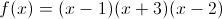#### Question 2 2. What is the rate of change at the point A in the graph below (for y in relation to x)?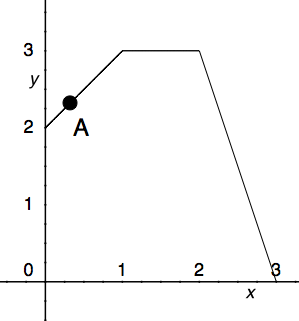#### Question 4 4. Consider the function f(x) given below. According to Rolle's theorem, between x=0 and x=pi, for how many values of x is the instantaneous rate of change equal to 0?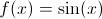#### Question 5 5. What is the rate of change at the point B in the graph below (for y in relation to x)? (In other words, what is the slope of the line from x = 2 to x = 3?)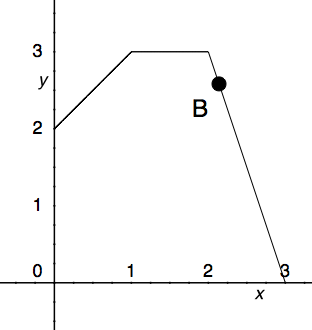### Page 2

#### Question 6 6. What is the average rate of change for the interval with endpoints A and B?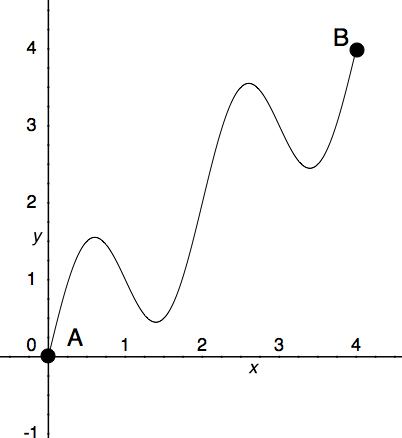#### Question 8 8. Which of the following is a local minimum?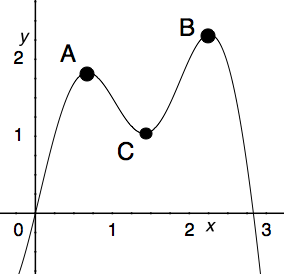#### Question 9 9. Where is the global maximum for the function y=f(x) below between x=0 and x=2?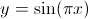#### Question 10 10. What is the rate of change at the point A in the graph below (for y in relation to x)?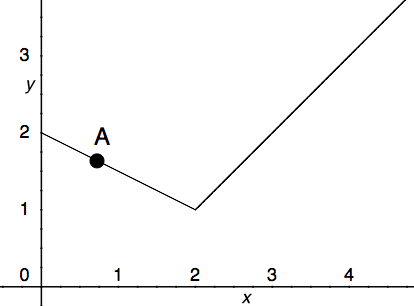### Page 3

#### Question 12 12. What is the approximate instantaneous rate of change at point C?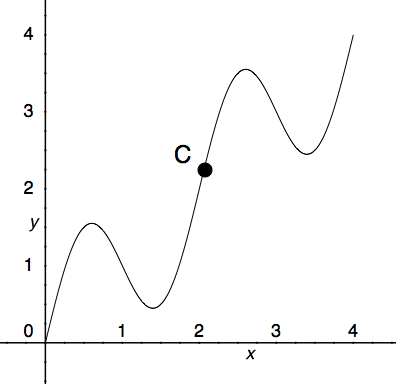#### Question 13 13. What is the rate of change at the point B in the graph below (for y in relation to x)?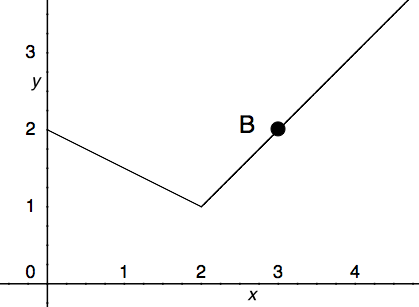### Page 4

#### Question 17 17. According to the mean value theorem, at how many points will the instantaneous rate of change equal the average rate of change between x = 1 and x = 3 on the following graph?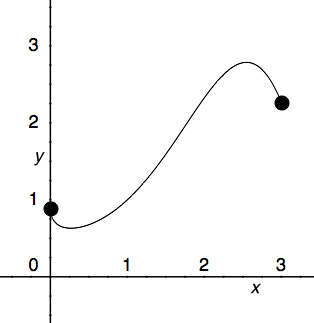#### Question 18 18. Which of the following BEST describes point B?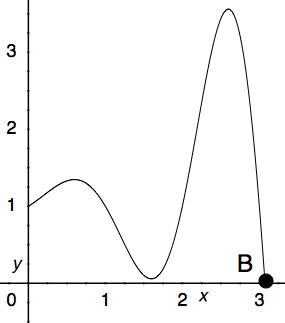#### Question 19 19. What is the rate of change at the point E in the graph below (for y in relation to x)?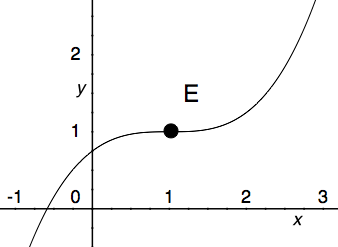### Page 5

#### Question 22 22. What is value of y at the finite local maximum for the function y=f(x) below?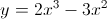#### Question 23 23. Over the region shown, the global minimum is at the point#### Question 24 24. What is the global minimum for the function y=f(x) below between x=0 and x=2?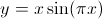#### Question 25 25. What is the global minimum for the function y=f(x) below between x=0 and x=2?### Page 6

#### Question 27 27. Which of the following BEST describes point C?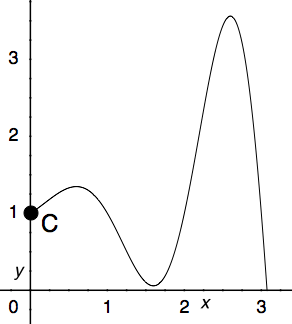#### Question 28 28. Which of the following BEST describes point A?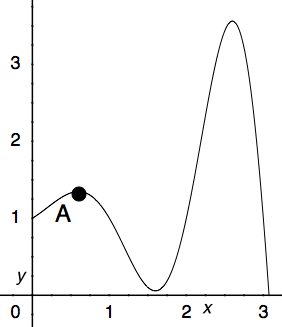#### Question 29 29. Which point on the following graph satisfies the mean value theorem between x = 0 and x = 3?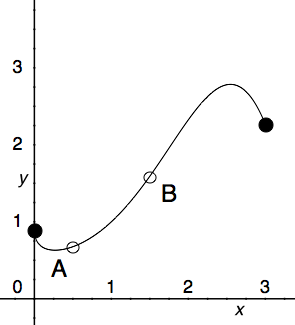#### Question 30 30. Consider the graph below. Between x=C and x=-C, for any value of C, will there be a point where the instantaneous rate of change is 0?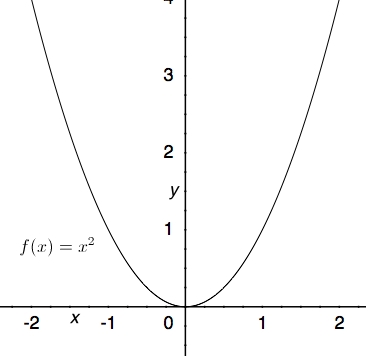#### Differentiable Functions & Min-Max Problems Chapter Exam Instructions

Choose your answers to the questions and click 'Next' to see the next set of questions. You can skip questions if you would like and come back to them later with the yellow "Go To First Skipped Question" button. When you have completed the practice exam, a green submit button will appear. Click it to see your results. Good luck!

Support